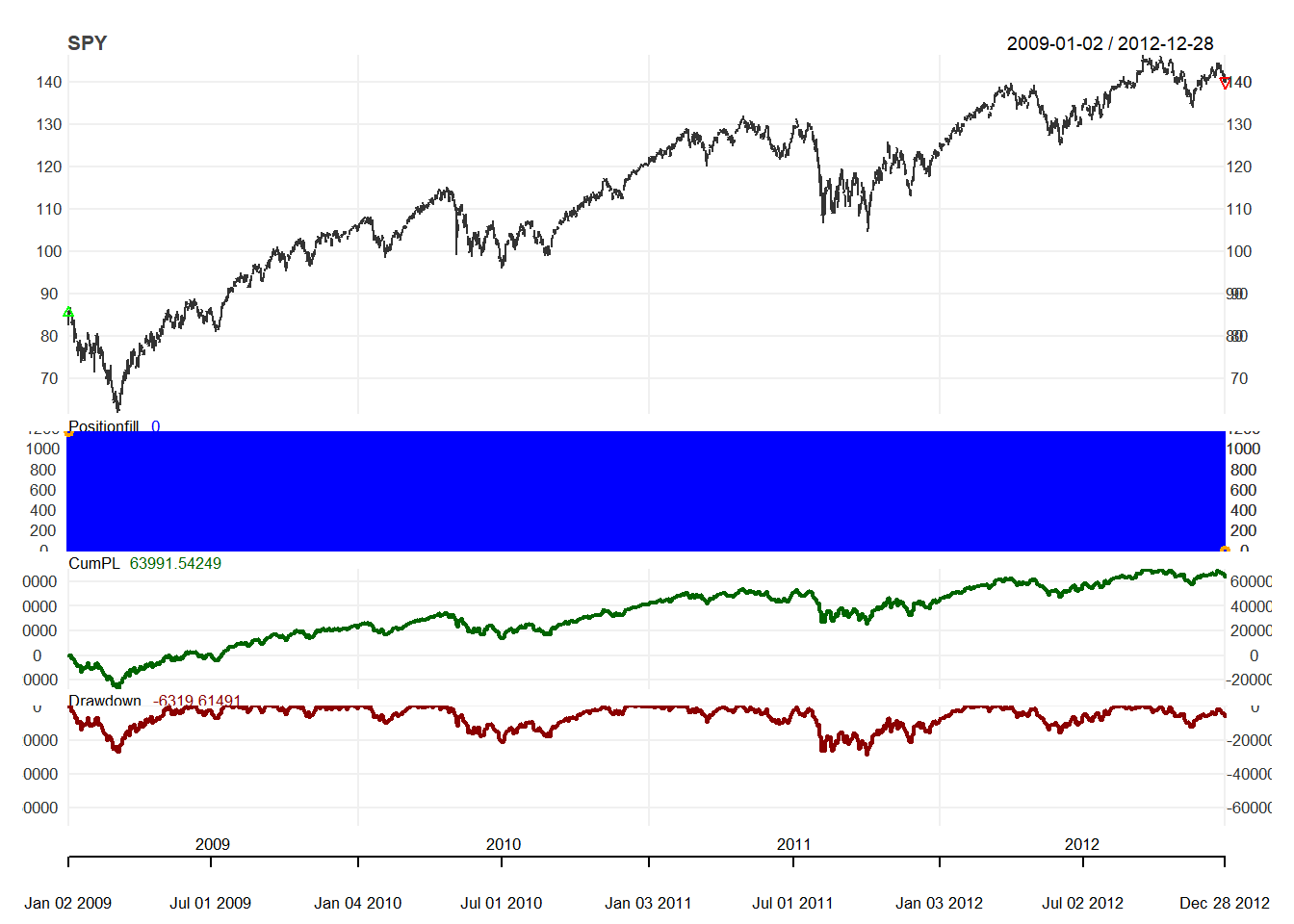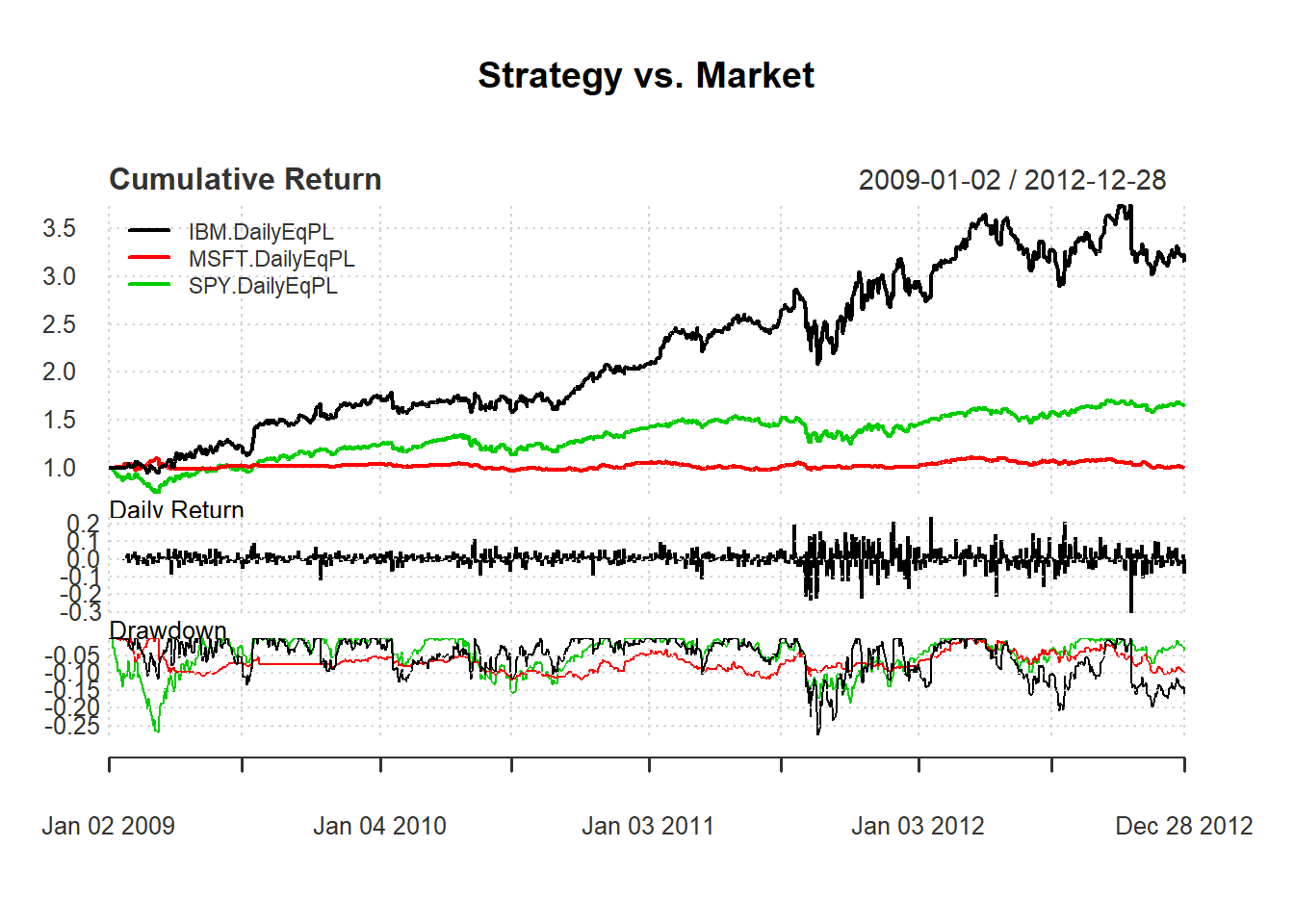We want to compare buy and hold strategy.

We first get the data

getSymbols("SPY", from=from, to=to, adjust=TRUE)

### 9.4.1 Step 1: Initialization

rm.strat("buyHold")

#Initial Setup
initDate = initDate, initEq = initEq)

### 9.4.2 Steps 2-4: Applying trading rule

FirstDate <- first(time(SPY))
# Enter order on the first date
UnitSize = as.numeric(trunc(equity/FirstPrice))
TxnDate = BuyDate, TxnPrice = FirstPrice,
TxnQty = UnitSize, TxnFees = 0)

We first add the transaction to sell at the end:

LastDate <- last(time(SPY))
# Exit order on the Last Date
LastPrice <- as.numeric(Cl(SPY[LastDate,]))
TxnDate = LastDate, TxnPrice = LastPrice,
TxnQty = -UnitSize , TxnFees = 0)

### 9.4.3 Step 5: Evaluation

We update our portfolio, account, and equity:

updatePortf(Portfolio = "buyHold")
updateEndEq(Account = "buyHold")

chart.Posn("buyHold", Symbol = "SPY")# Compare strategy and market
main = "Strategy vs. Market")